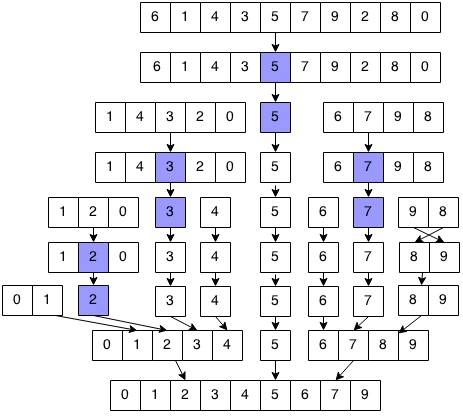# Complete algorithm of Quick Sort in Data Structure

In this article, we are going to learn about quick sort, how it works and its algorithm?
Submitted by Manu Jemini, on January 15, 2018

Quicksort is one the very popular sorting algorithm or technique to sort a collection of data. Quicksort is better because of few decent reasons.

1. It does not need any temporary storing memory; which means if you don’t need to invest any more storage capacity during the process. It makes sense when your data is quite large.
2. It is very fast because it uses divide and conquers. In this algorithm, we choose a pivot and divide it into two sub-arrays and then repeat the process again.The Above example shows it clearly that first choose the pivot which is 5 in the array 6 1 4 3 5 7 9 2 8 0. Now start from the first index and check if it’s greater than the pivot, if you found a greater element which 6 in our case, 6 1 4 3 5 7 9 2 8 0. We will replace it with the element which is lower than the pivot and is in the right side of the pivot or the pivot itself in some case.

When all the elements which are smaller than the pivot are on the left side and all the elements which are bigger than the pivot are on the right side, at that point we call the Quicksort function again with the different indices.

This approach makes it possible to process in the sub-array without conflict the other elements of the main array.

## Algorithm of Quicksort:

```quick(arr,first,last)

arr: is an array contains last elements
piv: contains pivot key
low/first: holds index of  lower bound
high/last: holds index of upper bound

step 1:[initialization]
set	low=first
set high=last
[Take the mid element of sublist as piv]
set piv=arr[(first+last)/2]

[Loop till pivot is placed at proper place in the sublist]

step 2: repeat step (3) to step (5) while low<=high

step 3:[Compare from  left side]
repeat step (i) 	while( arr[low]< piv )
i) set low=low+1
[end of step 3 loop]

step 4:[Compare from  right side]
repeat step (i)   while( arr[high]> piv )
i)	set high=high -1
[end of step 4 loop]

step 5:[swaping]
if( low<=high ) then
set temp=arr[low]
set arr[low]=arr[high]
set arr[high]=temp
set low=low+1
set high=high-1
[end of if statement]
[end of step (2) while loop]

step 6:[apply same process on sublist]
if(first<high) then
call quick(arr,first,high)
[end of if statement]
if(low<last) then
quick(arr,low,last)
[end of if statement]

step 7 return
```